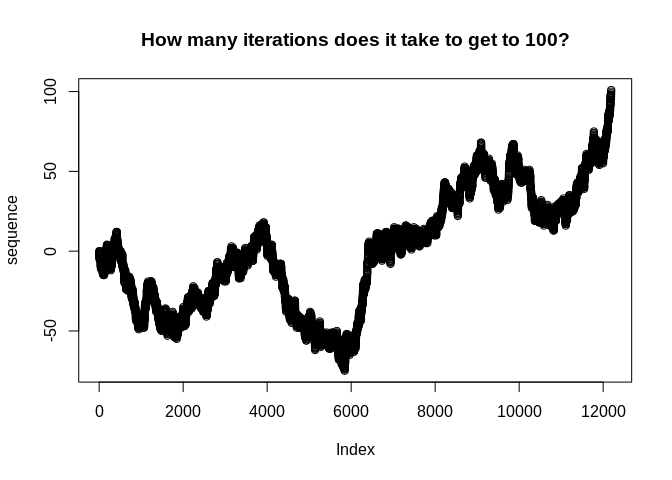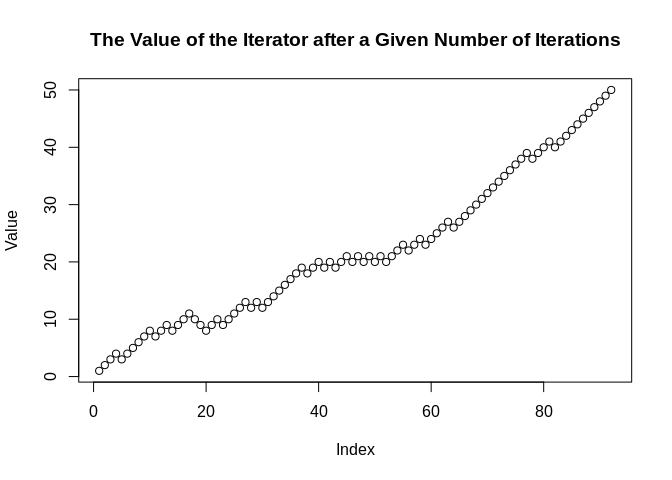The {peruse} package is aimed at making it easier to generate irregular sequences that are difficult to generate with existing tools.

The heart of {peruse} is the S3 class Iterator. An Iterator allows the user to write an arbitrary R expression that returns the next element of a sequence of R objects. It then saves the state of the Iterator, meaning the next time evaluation is invoked, the initial state will be the result of the previous iteration. This is most useful for generating recursive sequences, those where each iteration depends on previous ones.

The package also provides a simple, tidy API for set building, allowing the user to generate a set consisting of the elements of a vector that meet specific criteria. This can either return a vector consisting of all the chosen elements or it can return an Iterator that lazily generates the chosen elements.

At the end of this document, there is a tutorial for metaprogramming (that is, programmatically generating code) with Iterators.

## Installation

You can install the released version of peruse from CRAN with:

install.packages("peruse")

And the development version from GitHub with:

# install.packages("devtools")
devtools::install_github("jacgoldsm/peruse")

## Example

### Negative Binomial Investigation

Suppose we want to investigate the question of how many trials it takes for a random walk with drift to reach a given threshold. We know that this would follow a Negative Binomial distribution, but how could we use the Iterator to look at this empirically in a way that easily allows us to adjust the drift term and see how the result changes? We might do something like this:

p_success <- 0.5
threshold <- 100

iter <- Iterator({
set.seed(seeds[.iter])
n <- n + sample(c(1,-1), 1, prob = c(p_success, 1 - p_success))
},
list(n = 0, seeds = 1000:1e5),
n)
sequence <- yield_while(iter, n <= threshold)

plot(sequence, main = "How many iterations does it take to get to 100?")How would we apply this same function to a grid of probabilities? We could do something like this:

probs <- seq(0.5,0.95, by = 0.01)
num_iter <- rep(NA, length(probs))
threshold <- 20
seeds <- 1000:1e6

for (i in seq_along(probs)) {
iter <- Iterator({
set.seed(seeds[.iter])
n <- n + sample(c(1,-1), 1, prob = c(!! probs[i], 1 - !! probs[i]))
},
list(n = 0),
yield = n)

num_iter[i] <- length(yield_while(iter, n <= threshold))
}

plot(x = probs,
y = log(num_iter),
main = "Probability of Success vs How long it takes to get to 20 (Log Scale)",
xlab = "Probability of Success",
ylab = "Log Number of Iterations")Alternatively, using functional programming:

make <- function(p) {
iter <- Iterator({
set.seed(seeds[.iter])
n <- n + sample(c(1,-1), 1, prob = c(!! p, 1 - !! p))
},
list(n = 0),
yield = n)

length(yield_while(iter, n <= threshold))
}

num_iter <- sapply(seq(0.5,0.95, by = 0.01), make)

This illustrates a few useful features of Iterators:

• We can use environment variables in either our expression or our while condition to represent constants. In this case, threshold doesn’t change between iterations or between parameters. If you are creating many Iterators, it can be faster to use environment variables, since you don’t have to make a new object for each new Iterator.

• We can use the forcing operators from rlang (!!) to force evaluation of arguments in place, in this case substituting the expression of probs[i] with the value of probs[i] (see the end of this document for a tutorial on metaprogramming with Iterators).

• We can refer to the current iteration number in yield_while(), yield_more(), or their silent variants with the variable .iter.

### Collatz Sequence

A Collatz sequence is a particular sequence of natural numbers that mathematicians think always reaches 1 at some point, no matter the starting point. We can’t prove that one way or the other, but we can create an Iterator that lazily generates a Collatz sequence until it reaches 1:

library(peruse)

# Collatz generator starting at 50
collatz <- Iterator({
if (n %% 2 == 0) n <- n / 2 else n <- n*3 + 1
},
initial = list(n = 50),
yield = n)

yield_while(collatz, n != 1L)
#>   25 76 38 19 58 29 88 44 22 11 34 17 52 26 13 40 20 10  5 16  8  4  2  1

### Random Walk with Drift

Random Walks, with or without drift, are one of the most commonly used type of stochastic processes. How can we simulate one with {peruse}?

rwd <- Iterator({
n <- n + sample(c(-1L, 1L), size = 1L, prob = c(0.25, 0.75))
},
initial = list(n = 0),
yield = n)

Value <- yield_while(rwd, n != 50L & n != -50L)

plot(Value, main = "The Value of the Iterator after a Given Number of Iterations")Here, we can see that seq gets to 50 after about 100 iterations when it is weighted 3:1 odds in favor of adding 1 over adding -1 to the prior value.

## A Note

Helper functions yield_more(), yield_while(), move_more(), and move_while() behave mostly like syntactic sugar for explicit loops. So,

it <- Iterator(x <- x + 1L, list(x = 0), x)
it2 <- clone(it)
x <- numeric(100)
for (i in 1:100) {
x[i] <- yield_next(it)
}

will give the same result as

y <- yield_more(it2, 100)

However, doing it the latter way is significantly more efficient than the former. This is because a lot of the overhead only needs to be done once per call to yield. That means that a lot less has to be done on every iteration when you explicitly call yield_more().

This is even more true with yield_while(). Pretend we don’t know how long this Iterator will take to reach 10,000. Doing something like:

x <- numeric()
while (it$initial$x < 10000) {
x <- c(x, yield_next(it))
}

is very inefficient because it requires reallocating the vector on every iteration. On the other hand, the following is both easier to read and much more efficient:

y <- yield_while(it2, x < 10000)

Internally, yield_while() uses an efficient algorithm for resizing the result in linear amortized time. As a result, it will evaluate much faster.

### Primes

How about generating all the prime numbers between 1 and 100? We can easily do that with the set-builder API:

2:100 %>%
that_for_all(range(2, .x)) %>%
we_have(~.x %% .y)
#>    2  3  5  7 11 13 17 19 23 29 31 37 41 43 47 53 59 61 67 71 73 79 83 89 97

In the equation, we can reference the left-hand side of the equation with the positional variable .x, and the right-hand side (that is, the argument in that_for_all()) with .y. The equation can be anything recognized as a function by rlang::as_function().

But how about if we want to generate the first 100 prime numbers? We don’t know the range of values this should fall in (well, mathematicians do), so we can use laziness to our advantage:

primes <- 2:10000 %>%
that_for_all(range(2, .x)) %>%
we_have(~.x %% .y, "Iterator")

primes_2 <- clone(primes)

The first prime number is

yield_next(primes_2)
#>  2

And the first 100 are:

sequence <- yield_more(primes, 100)
sequence
#>      2   3   5   7  11  13  17  19  23  29  31  37  41  43  47  53  59  61
#>    67  71  73  79  83  89  97 101 103 107 109 113 127 131 137 139 149 151
#>   157 163 167 173 179 181 191 193 197 199 211 223 227 229 233 239 241 251
#>   257 263 269 271 277 281 283 293 307 311 313 317 331 337 347 349 353 359
#>   367 373 379 383 389 397 401 409 419 421 431 433 439 443 449 457 461 463
#>   467 479 487 491 499 503 509 521 523 541

Here, we use clone() to create an identical Iterator to primes that can be modified separately.

### One Exception

Ordinarily, Iterators work independently from the environment in which they were created. The one exception is when an Iterator is created from the template,

iterator <- .x %>% expression_with_.x %>% we_have(formula, "Iterator")

the variable expression_with_.x is turned into a quosure. That means that it will always be evaluated in the environment where iterator was made.

For a concrete example, consider:

offset <- 2
it <- 2:100 %>% that_for_all(range(offset, .x)) %>% we_have(~ .x %% .y, "Iterator")
fun <- function() {
offset <- 3
yield_while(it, !.finished) # print the whole sequence
}

fun()
#> (Note: result has reached end of sequence)
#>    2  3  5  7 11 13 17 19 23 29 31 37 41 43 47 53 59 61 67 71 73 79 83 89 97

We can see that the code does not select elements that are divisible by 2 but not any other numbers, as would be the case with offset equal to three. Our expression range(offset, .x) is evaluated in the global environment, not in the execution environment of fun().

# Citation

This software contains a modified version of a small piece of code from the purrr package, by Hadley Wickham, Lionel Henry, and RStudio, freely available under the MIT License.